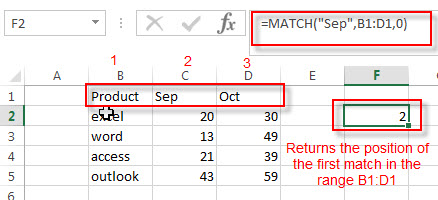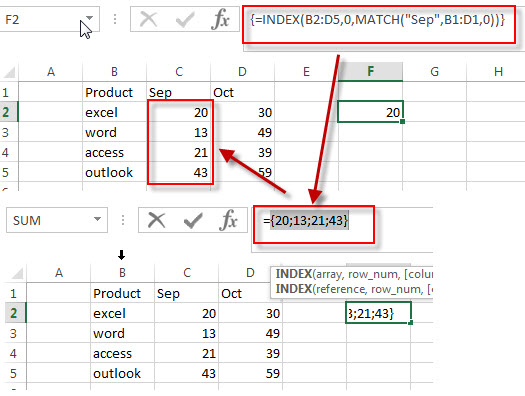# How to Extract the Entire Column of a Matched Value

In the previous post, we talked that how to lookup value and then returns entire rows of a matched value in a range. And this post will guide you how to lookup value and then return the entire column of the first matched value in a range in excel. How to use INDEX/MATCH to lookup entire column and retrieve the entire row.

## Lookup Entire Column

If you want to lookup value in a range and then retrieve the entire Column, you can use a combination of the INDEX function and the MATCH function to create a new excel formula.

For example, if you want to search “Sep” string in the range B1:D1, then return the entire column of a matched value in the range B2:D5, you can write down the following array formula:

`=INDEX(B2:D5,0,MATCH("Sep",B1:D1,0))`

Let’s see how this formula works:

=MATCH(“Sep”,B1:D1,0)The MATCH function returns the position of the lookup value “Sep” in the range B2:D1. The third argument is set to 0, it means that the MATCH function will search the first lookup value exactly.

=INDEX(B2:D5,0,MATCH(“Sep”,B1:D1,0))The second argument is specified to 0 in the INDEX function, it means that the row number is set to 0, it will return all the values in the entire column and the position number of column is returned by the MATCH function. So this formula returns the values of the COLUMN 2 in the range B2:D5.

### Related Formulas

• Lookup Entire Row using INDEX/MATCH
If you want to lookup entire row and then return all values of the matched row, you can use a combination of the INDEX function and the MATCH function to create a new excel array formula.
• Lookup the Value with Multiple Criteria
If you want to lookup the value with multiple criteria in a range, you can use a combination with the INDEX function and MATCH function to create an array formula.…
• Lookup the Next Largest Value
If you want to get the next largest value in another column, you can use a combination of the INDEX function and the MATCH function to create an excel formula..
• Find the nth Smallest Value
You can use the SMALL function to get the 1st, 2nd, 3rd, or nth smallest value in an array or range. Also you can use the SMALL function within the INDEX function to extract the relative value of the same row…

### Related Functions

• Excel INDEX function
The Excel INDEX function returns a value from a table based on the index (row number and column number)The INDEX function is a build-in function in Microsoft Excel and it is categorized as a Lookup and Reference Function.The syntax of the INDEX function is as below:= INDEX (array, row_num,[column_num])…
• Excel MATCH function
The Excel MATCH function search a value in an array and returns the position of that item.The syntax of the MATCH function is as below:= MATCH  (lookup_value, lookup_array, [match_type])….